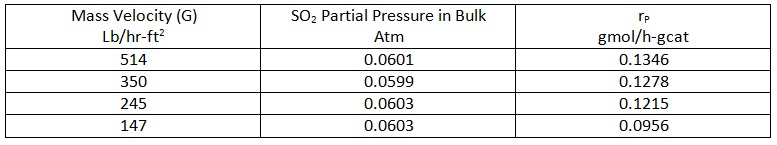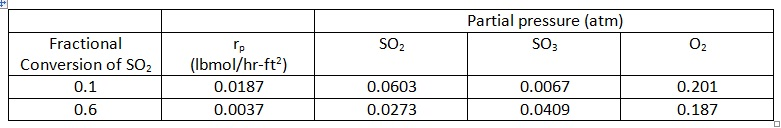+61-413 786 465

info@mywordsolution.com

## Engineering

 Civil Engineering Chemical Engineering Electrical & Electronics Mechanical Engineering Computer Engineering Engineering Mathematics MATLAB Other Engineering Digital Electronics Biochemical & Biotechnology

Film Mass Transport:

problem: Sulfur trioxide (SO3) is manufactured by the gas-phase oxidation of SO2 over a platinum catalyst:

SO2 + 1/2 O2 → SO3

The catalyst is a non-porous extrudate with the platinum deposited on the outside surface. The following data have been measured for the particle rate of reaction as a function of SO2 concentration in the bulk gas at 450 °CThe following data apply to this problem:

? B (void fraction) = 0.43
Catalyst = 1/8 x 1/8 inch (diameter x length) extrudates (Pt on surface only)
At (specific external surface area of catalyst) = 5.12 ft2/lb
DSO2/air = 1.1 ft2/h
μ air = 0.09 lb/hr-ft
ρair = 0.0304 lb/ft3

Without calculating anything, what can you tell about the importance of film mass transport on this reaction? describe briefly.

problem: describe why mass transfer resistance reduces the global rate more at higher temperature than at lower temperature.  Assume no heat transfer resistances are present.

problem: A gas-phase catalytic reaction is taking place in a Packed Bed Reactor (PBR). The system is isothermal but film mass transfer resistances are important.

a) Would increasing the turbulence in the gas phase increase or decrease the global rate?

b) If the system is not isothermal and the reaction is exothermic would increasing the turbulence increase or decrease the global rate?

problem: Experimental global rate data for the oxidation of SO2 over a non-porous platinum catalyst are given in the table below for two levels of conversion of SO2.  Estimate the importance of film mass transport from these data by calculating the concentration difference (for SO2) between the bulk gas and the catalyst surface.

DATA:

a) Packed-bed reactor (PBR); catalyst consists of 1/8 x 1/8-inch (radius x length) tablets
b) Packing void fraction (εB) = 0.36
c) Superficial mass velocity (G) = 147 lb/hr-ft2
d) Pressure = 790 mm Hg; Temperature (assume isothermal) = 480 °C
e) Bulk gas concentration: 6.42 mol% SO2 and 93.58 mol% air
f) Specific external surface area of catalyst (am) = 5.12 ft2/lbrp = particle rate of reaction (rate per unit external surface area of catalyst)

problem: Cumene (C) is catalytically cracked to manufacture benzene (B) and propylene (P). The following non-stoichiometric equation illustrates the chemistry:

C → B + P

Typical operating conditions for this reaction are a temperature and total pressure of 362 °C and 1.0 atm. respectively.  A measurement of the global rate of reaction was made in the laboratory, resulting in the following value for the rate of disappearance of cumene:

rp = 76.5 kmol/m2 – h

From the data supplied, is there any evidence of either heat or mass transfer limitations for this reaction? Be as quantitative as possible in your explanation.

Assumptions:

The catalyst particle is non-porous. All thermo physical properties (density, viscosity, thermal conductivity, etc.) of the bulk gas and gas in the film can be assumed to be constant.

Data:

Average MW of gas = 34.37 kg/kmol
Gas density = 0.66 kg/m3
Gas viscosity = 0.094 kg/m – h
Gas thermal conductivity = 0.037 kcal/m – h - °C
Gas heat capacity = 33.0 kcal/kg – °C
G (mass velocity) = 56,470 kg/m2 – h
at = am = 45 m2/kg cat (specific external surface area of catalyst)
εB (bed void fraction) = 0.5
dp (catalyst particle diameter, equivalent sphere) = 0.1 cm
ΔHr (heat of reaction) = +41,816 kcal/kmol (endothermic)
Ea (activation energy for reaction) = 40 kcal/gmol
ρB (catalyst bulk density) = 5x105 g/m3
Sc (Schmidt number) = 1.483

Chemical Engineering, Engineering

• Category:- Chemical Engineering
• Reference No.:- M9305

Have any Question?

## Related Questions in Chemical Engineering

### 500 kghr of steam drives turbine the steam enters the

500 kg/hr of steam drives turbine. the steam enters the turbine at 44 atm and 450 c at a velocity of 60 m/sec and leavects at point 5 m below the turbine inlet at atmospheric pressure and velocity of 360 m/sec. the turbi ...

### Problem 1 review the concept of fluxeswhat are the mass

Problem 1: Review the concept of fluxes What are the mass flow rates through each of the surfaces described below and shown in the figure? For some of these cases, you are free to justify your answer with words, rather t ...

### Biomass and biorefineries executive report assignment -you

Biomass and Biorefineries Executive Report Assignment - You are employed in the R&D facility of a large publically listed ago-forestry company that has been highly profitable in the past. There are early warning signs of ...

• 13,132 Experts

## Looking for Assignment Help?

Start excelling in your Courses, Get help with Assignment

Write us your full requirement for evaluation and you will receive response within 20 minutes turnaround time.

### Why might a bank avoid the use of interest rate swaps even

Why might a bank avoid the use of interest rate swaps, even when the institution is exposed to significant interest rate

### Describe the difference between zero coupon bonds and

Describe the difference between zero coupon bonds and coupon bonds. Under what conditions will a coupon bond sell at a p

### Compute the present value of an annuity of 880 per year

Compute the present value of an annuity of \$ 880 per year for 16 years, given a discount rate of 6 percent per annum. As

### Compute the present value of an 1150 payment made in ten

Compute the present value of an \$1,150 payment made in ten years when the discount rate is 12 percent. (Do not round int

### Compute the present value of an annuity of 699 per year

Compute the present value of an annuity of \$ 699 per year for 19 years, given a discount rate of 6 percent per annum. As